# Properties of Polyhedral Oligomeric Silsesquioxane-Modified Cellulose Insulation Paper with Different Number of Phenyls

Properties of Polyhedral Oligomeric Silsesquioxane-Modified Cellulose Insulation Paper with Different Number of Phenyls
Electric Power Research Institute, State Grid Chongqing Electric Power Company, Chongqing 401123, China

University Library, Southwest University, Chongqing 400715, China

Corresponding Author Email:
swulj@swu.edu.cn
Page:
383-389
|
DOI:
https://doi.org/10.18280/ijht.390207
3 January 2021
|
Accepted:
9 March 2021
|
Published:
30 April 2021
| Citation

OPEN ACCESS

Abstract:

Polyhedral oligomeric silsesquioxane (POSS) has a total of eight substituents. The number of substituents determines the modification effect of POSS. Through simulation of molecular dynamics, this paper explores how the number of substituents affects the POSS-modified cellulose insulation paper. Specifically, the mechanical properties, thermal stability and polarizability were calculated for cellulose models with 1-phenyl POSS, 2-phenyl POSS, 3-phenyl POSS, 4-phenyl POSS, 5-phenyl POSS, 6-phenyl POSS, 7-phenyl POSS, and 8-phenyl POSS, respectively. The results show that the cellulose model modified by 6-phenyl POSS achieved better effect than the other seven models, as evidenced by its distinctively large bulk modulus, shear modulus, and elastic modulus. Besides, the cellulose model modified by 6-phenyl POSS had the most stable and the minimum mean square displacement (MSD). This is because the mechanical properties of the insulation paper are improved, as the motion of cellulose chains is inhibited by the nano-size effect of POSS and the effect of phenyls. Further, the cellulose model modified by 6-phenyl POSS also had the smallest polarizability among the modified models. Therefore, the 6-phenyl POSS-modified cellulose model can effectively reduce the polarizability of cellulose, uniform the electric field distribution in oil-paper insulation system, and thus enhance the insulation property of transformers.

Keywords:

cellulose insulation paper, polyhedral oligomeric silsesquioxane (POSS), mechanical properties, thermal stability, polarizability

1. Introduction

The development of power network has posed severe challenges to the oil-paper insulation system in power transformers. During the long-term operation, the mechanical properties and thermal stability of the transformers will be undermined by the thermal aging of the insulation paper, i.e., the decline of insulation performance [1-5]. In fact, the performance of a power transformer hinges directly on the property of insulation paper [6-10]. A popular way to improve the property of insulation paper is to modify cellulose insulation paper with nano-technology.

Nanoparticle modified insulation paper has been successfully applied in many fields [11-13]. Liao et al.  modified insulation paper with nano-TiO2 of different mass fractions, immersed the modified insulation paper in the insulating oil in vacuum, and tested that nano-TiO2 can significantly improve the strength of the power-frequency breakdown field for the insulation paper: when the mass fraction of nano-TiO2 was 3%, the breakdown field strength increased by the greatest extent, while the dielectric constant, dielectric loss, and conductivity of the insulation paper reached the minimum. Through deposition and chemical modification, Rodríguez-Uicab et al.  added multiwalled carbon nanotubes to the surface of meta-aramid fiber, and verified the effectiveness of the modification method by scanning electron microscopy (SEM), Raman spectroscopy and X-ray photoelectron spectroscopy (XPS). Chen  modified the insulating oil with nano-TiO2, nano-Fe3O4, and nano-Al2O3, separately, and then impregnated the insulation paper in each nano-modified insulating oil; every modified insulation paper saw improvement in the power-frequency partial discharge inception voltages and surface flashover voltages, among which the improvement by nano-TiO2 modification was the most obvious. Tang et al.  modified meta-aramid insulation paper with polyphenylsilsesquioxane (PPSQ), verified improved thermal stability of the modified insulation paper through experiments and molecular simulation, and analyzed the inner mechanism of the improvement based on simulation results.

The above literature of modified cellulose insulation paper suggests that nanoparticle modification is an effective way to improve the thermal stability and mechanical properties of the insulation paper. Polyhedral oligomeric silsesquioxane (POSS)  is a typical organic-inorganic hybrid compound with nanoscale molecules. The POSS has a cage-shaped inorganic core composed of alternatively linked Si-O skeleton, and involves eight substitutable groups. Any change to the substituents could alter the modification results . However, there is little report on how the number of substituents affects the cellulose insulation paper modified with the POSS. Peng et al.  prepared poly-silsesquioxane containing different functional groups by catalytic hydrolysis and condensation, tested the thermal stability of poly-silsesquioxane through thermogravimetric analysis (TGA), and demonstrated that octaphenylsilsesquioxane (OPPOSS) has the best thermal stability. Therefore, this paper decides to explore the thermodynamic properties of POSS-modified cellulose insulation paper with different number of phenyl substituents.

2. Methodology

2.1 Model construction

This paper probes into the influence of the number of substituents on POSS-modified cellulose insulation paper through molecular dynamics simulation. Figure 1(a) shows the 1-phenyl POSS model. Previous studies have shown that fiber models have little mechanical and chemical differences in the amorphous-region, as long as the polymerization degree of fiber molecules is smaller than 10 . Tang et al.  tested the cellulose with polymerization degree of 20, and found that the tested mechanical properties are consistent with simulation results.

To reduce model size and speed up calculation, this paper establishes a model with the fiber molecules of the polymerization degree of 10 (Figure 1(b)). The silicon atoms, oxygen atoms, hydrogen atoms, and gray atoms are silicon, oxygen, hydrogen, and carbon, respectively. After model optimization, Amorphous_Cell module was called to build a periodic amorphous model for the blending of cellulose and POSS with different number of phenyl substituents, following the method proposed by Theodorou and Suter . The initial density of the model was set to 1.2g/cm3.

Wang et al.  held that, under the Condensed-phase Optimized Molecular Potential for Atomistic Simulation Studies (COMPASS) stand, the steady-state density of cellulose is close to the real value, reflecting the real state of cellulose. Therefore, this paper adopts the COMPASS stand, and modifies cellulose insulation paper with 1-phenyl POSS, 2-phenyl POSS, 3-phenyl POSS, 4-phenyl POSS, 5-phenyl POSS, 6-phenyl POSS, 7-phenyl POSS, and 8-phenyl POSS, separately. Among them, the 1-phenyl POSS, 4-phenyl POSS, and 8-phenyl POSS models are shown in Figure 2.

## 1.png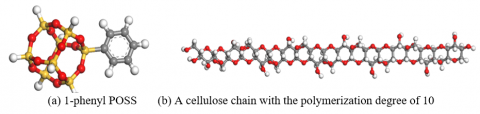Figure 1. Sketch diagram of the established model

## 2.png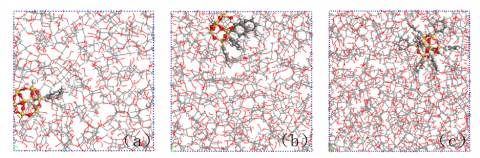Figure 2. (a) Cellulose model modified with 1-phenyl POSS (b) Cellulose model modified with 4-phenyl POSS (c) Cellulose model modified with 8-phenyl POSS

2.2 Parameter setting

To keep the model in a stable state, the structure of each model was optimized by 10,000 steps, and the rationality of the model structure was estimated by adjusting the system energy and temperature and annealing them by ten 300-900K cycles with an interval of 50K in constant-temperature, constant-volume ensemble (NVT), before the calculation of molecular dynamics. If the model was found to be unreasonable, optimization and annealing would be carried out again; if the model was found to be reasonable, the model with the minimum energy would be selected, and the dynamic calculation of 500ps would be conducted under the constant-temperature, constant-pressure ensemble (NPT). To increase the model density close to the experimental value, the pressure was set to 1GPa. Then, the dynamic calculation of 500ps was performed under NVT to make the model fully balanced, and continued under NPT to ensure the model performs similarly as the real material. Under the temperature of 343K and the atmospheric pressure, the dynamic information was collected every 0.5ps to provide the trajectory information for the subsequent performance calculation. The COMPASS force field was adopted throughout the process.

3. Calculation

3.1 Mechanical properties

The polycrystalline substance composed of randomly oriented microcrystals is statistically isotropic. Its mechanical parameters can be obtained by Reuss average method  for isotropic materials. Specifically, the volume modulus (K), shear modulus (G), elastic modulus (E), Poisson’s ratio (ν) and Cauchy pressures (C12-C44) can be respectively calculated by:

$K=[3(a+2 b)]^{-1}$    (1)

$G=5[(4 a-4 b+3 c)]^{-1}$      (2)

$E=2 G(1+v)=3 K(1-2 v)$      (3)

$C_{44}=\frac{1}{2}\left(C_{11}-C_{12}\right)$      (4)

where,

$a=\frac{1}{3}\left(S_{11}+S_{22}+S_{33}\right)$    (5)

$b=\frac{1}{3}\left(S_{12}+S_{23}+S_{31}\right)$      (6)

$c=\frac{1}{3}\left(S_{44}+S_{55}+S_{66}\right)$      (7)

Note that $C_{i j} \text { is a } 6 \times 6$ elastic coefficient matrix; a, b, and c represent the column vectors formed by the side length at the deformation time. The inverse matrix of $C_{i j}$ needs to be computed to drive matrix $S_{i j}$.

In elastic mechanics, the elastic modulus (E), i.e., the ratio of stress to strain acting on the material, is positively correlated with the material’s ability to resist deformation. The shear modulus (G), i.e., the ratio of shear stress to strain, is positively correlated with the material’s resistance to shear stress; it is also known as rigid modulus. The volume modulus (K), i.e., the relation between the volume strain and the mean stress of the material, is positively correlated with the material’s incompressibility. The Poisson’s ratio (ν), i.e., the ratio of transverse strain to longitudinal strain, is positively correlated with the material’s plasticity; it reflects the relationship between deformation in two directions, and has little to do with the degree of deformation. The Cauchy pressures (C12-C44) reflects the ductility of the material; if the value is positive, the material has good ductility; if the value is negative, the material is highly brittle.

3.2 Mean square displacement (MSD)

The MSD, i.e., the mean particle displacement, is often used to characterize the motion performance of particles . This index is positively correlated with the intensity of chain motion, and the thermal instability of cellulose. The MSD value can be calculated by:

$M S D=\left\langle\left|r_{i}(t)-r_{i}(0)\right|\right\rangle^{2}$    (8)

where, $r_{i}(t) \text { and } r_{i}(0)$ are the position vectors of a molecule or an atom at time t or initial time i, respectively.

3.3 Free volume

The cellulose material consists of molecules and cavities. The volume not occupied by molecules is called the free volume. The free volume fraction can be defined as the ratio of the free volume to the total volume of the material:

$F F V=\frac{V_{f}}{V_{f}+V_{O}}$    (9)

where, FFV is free volume fraction; $V_{f}$ is free volume; $V_{O}$ is occupied volume.

3.4 Dipole moments and polarization

The dielectric constant, a commonly used metric of the insulating properties of dielectric insulation materials, is mainly determined by the mean polarizability . The polarizability of a polymer can be derived from the dipole moments per unit volume. The molecular dipole moments in the directions of x, y and z can be obtained by analyzing the dynamic trajectory files through molecular dynamics simulation.

4. Results and Discussion

4.1 Mechanical properties

Figure 3 presents the mechanical properties of each modified cellulose model. The relevant mechanical parameters were calculated through molecular dynamics simulation.

## 3.png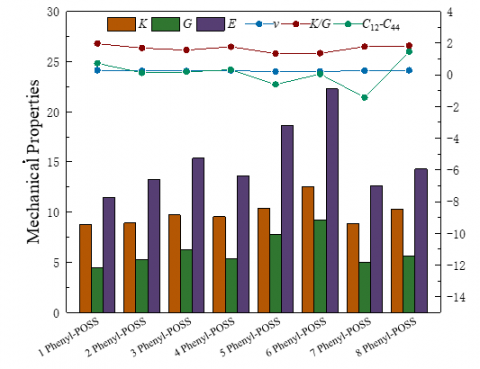Figure 3. Mechanical properties of each modified cellulose model

It can be seen from Figure 3 that, the 6-phenyl POSS-modified cellulose model achieved much higher bulk modulus (K), shear modulus (G), and elastic modulus (E) than the other modified cellulose models, while having similar Poisson's ratio (ν), bulk modulus, and shear modulus (K/G) as the other models. The results show that the 6-phenyl POSS-modified cellulose model has the best modification effect, as evidenced by its extraordinary anti-deformation ability and incompressibility. The main reason for the good effect is as follows:

The highly stable Si-O bond and cage structure of POSS improve the mechanical properties of the cellulose. The POSS has the advantages of stable structure and large volume. In molecular dynamics simulation, the angle of Si-O bond only changes slightly, and the three-dimensional (3D) spatial structure of POSS basically remains stable. This means the motion of POSS needs to overcome great forces. In addition, the aromatic structure of phenyl has good heat resistance and rigidity. Thus, the addition of phenyl improves the mechanical properties of the modified model. Among the eight models, the cellulose model modified by the 6-phenyl POSS witnesses the smoothest chain motion. The additive nano-particles reduces the gap between the cellulose molecular chains, inhibits the motion of these chains, and reduces the degree of motion of the cellulose molecules, giving the modified model outstanding mechanical properties.

4.2 MSD

To study the chain motion in each modified model, the MSD of each model was calculated by the Forcite module for 200ps. The calculated results are shown in Figure 4. Note that the simulation temperature was kept at 343K to mimic the working state of cellulose insulation paper in power transformers.

As shown in Figure 4, the MSD curve of 6-phenyl POSS-modified cellulose model was smoother than any other model, while that of 5-phenyl POSS and 8-phenyl POSS modified cellulose models was steep, with a large slope. Hence, the latter two models have strong molecular chain motions. During the simulation, the MSD of the fiber chains in the 6-phenyl POSS-modified cellulose model fluctuated between 0 and 1.01 Å, that of the 5-phenyl POSS-modified cellulose model fell within 0 and 1.64Å, and that in the 8-phenyl POSS-modified cellulose model ranged from 0 to 1.55Å. Overall, the 6-phenyl POSS effectively restrains the motion of cellulose chains, and leads to the best modification effect. Therefore, 6-phenyl POSS has the strongest binding effect on cellulose molecular chains.

## 4.png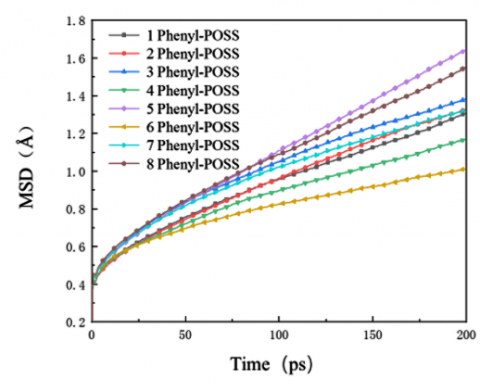Figure 4. MSD of each modified cellulose model

4.3 Free volume

By analyzing the trajectory files, the free volume fraction of each modified cellulose model was obtained. The relevant results are reported in Table 1 and Figure 5, where the blue and gray colors represent the free volume and the occupied volume, respectively.

Table 1. Free volume fraction of each modified cellulose model

 Model 1-phenyl POSS 2-phenyl POSS 3-phenyl POSS 4-phenyl POSS 5 phenyl POSS 6-phenyl POSS 7 phenyl POSS 8-phenyl POSS Occupied/Å³ 16,812.59 23,573.68 21,901.16 23,652.63 26,958.92 28,801.03 32,133.99 33,715.99 Free/Å³ 2,080.16 3,077.36 3,533.42 2,776.27 3,957.15 3,669.92 4,267.97 5,178.71 FFV/% 11.01 11.55 13.89 10.50 12.80 11.30 11.72 13.31

## 5.png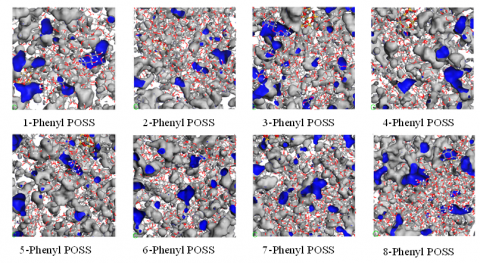Figure 5. Free volume of each modified cellulose model

From Figure 5 and Table 1, it can be observed that 4-phenyl POSS-modified cellulose model had the smallest free volume fraction, while of 5-phenyl POSS and 8-phenyl POSS had comparatively large free volume fractions. This echoes with the previous results on MSD. Under the nano-size effect of POSS, the remaining cellulose chains bonded more tightly, resulting in closer intermolecular connection; the free volume is also reduced as some spaces are occupied by the molecular chains.

Notably, the free volume of the 6-phenyl POSS model was well-distributed, and relatively small within per unit volume. The cellulose molecular chains are compressed, narrowing the gap between them. As a result, the structure of the 6-phenyl POSS model is highly stable. The molecular chain motion of the model is limited, making the MSD curve more smooth.

4.4 Dipole moments and polarization

The dipole moments of 1-phenyl POSS, 2-phenyl POSS, 3-phenyl POSS, 4-phenyl POSS, 5-phenyl POSS, 6-phenyl POSS, 7-phenyl POSS, and 8-phenyl POSS modified cellulose models in the three directions were calculated, and the results are shown in Figure 6.

The total dipole moment was calculated by the vector sum of dipole moments in the three directions, and then the mean polarizability was obtained by dividing the total dipole moment by the total volume. The results are shown in Table 2.

## 6.png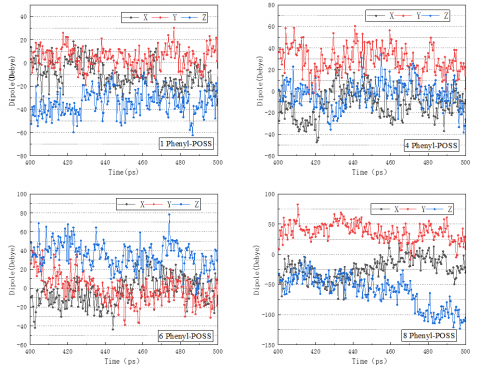Figure 6. Dipole moments of each modified cellulose model

Table 2. Polarization of each modified cellulose model

 The Model Total volume (Å3) Total Dipole Moment (D) Polarizability (D·Å-3) 1-phenyl POSS 17,070.5 39.12 2.29×10-3 2-phenyl POSS 24,079.6 36.65 1.52×10-3 3-phenyl POSS 22,465.8 42.72 1.90×10-3 4-phenyl POSS 24,175.3 37.32 1.54×10-3 5-phenyl POSS 27,514.9 90.31 3.28×10-3 6-phenyl POSS 29,135.0 41.67 1.43×10-3 7-phenyl POSS 32,739.7 68.02 2.08×10-3 8-phenyl POSS 34,609.0 81.49 2.36×10-3

From Figure 6 and Table 2, the POSS with different number of phenyl substituents influenced the polarization of modified cellulose models. Comparatively, the 6-phenyl POSS-modified model realized the lowest polarizability. Because of the low dielectric constant of POSS molecules, the polarization of the modified cellulose model decreased with the addition of POSS. In addition, the molecular weight of POSS is much larger than that of cellulose chain, making it difficult to flip the dipole bonded by nanoparticles in the modified model. The higher the content of POSS, the more the bound dipoles, and less polarized the model. Further, owing to the cage structure of POSS and the addition of phenyls, the porosity and air content of the modified model increased, and the polarization of cellulose decreased, with the addition of POSS.

5. Conclusions

Through molecular dynamics simulation, this paper studies the properties of the cellulose insulation paper modified by POSS with different number of phenyls, and draws the following conclusions:

(1) The number of phenyl substituents affects the mechanical properties and thermal stability of POSS-modified cellulose insulation paper.

(2) The 6-phenyl POSS-modified cellulose model shows the best mechanical properties and thermal stability, the least MSD, and the largest bulk modulus, shear modulus and elastic modulus, among all POSS-modified cellulose models.

(3) The 6-phenyl POSS-modified cellulose model also achieves the lowest polarizability among all models. This model can effectively reduce the polarization of cellulose, optimize the distribution of electric field in oil-paper insulation system, and thus enhance the insulation of transformers.

References

 Zhang, Y., Li, Y., Zheng, H., Zhu, M., Liu, J., Yang, T., Zhang, C.H., Li, Y. (2020). Microscopic reaction mechanism of the production of methanol during the thermal aging of cellulosic insulating paper. Cellulose, 27(5): 2455-2467. https://doi.org/10.1007/s10570-019-02960-6

 Wang, J., Liao, R., Cheng, L., Cheng, Z. (2020). Effects of winding vibration on the mechanical-thermal aging properties of insulating paper. IEEE Access, 8: 67912-67920. https://doi.org/10.1109/ACCESS.2020.2984257

 Zhuravleva, N., Reznik, A., Tukacheva, A., Kiesewetter, D., Smirnova, E. (2016). The study of thermal aging components paper-impregnated insulation of power transformers. In 2016 IEEE NW Russia Young Researchers in Electrical and Electronic Engineering Conference (EIConRusNW), pp. 747-751. https://doi.org/10.1109/EIConRusNW.2016.7448288

 Zhu, X., Yin, Y., Wu, J., Wang, X. (2020). Study on aging characteristics of XLPE cable insulation based on quantum chemical calculation. IEEE Transactions on Dielectrics and Electrical Insulation, 27(6): 1942-1950. https://doi.org/10.1109/TDEI.2020.009025

 Wei, Y.H., Mu, H.B., Deng, J.B., Zhang, G.J. (2016). Effect of space charge on breakdown characteristics of aged oil-paper insulation under DC voltage. IEEE Transactions on Dielectrics and Electrical Insulation, 23(5): 3143-3150. https://doi.org/10.1109/TDEI.2016.7736879

 Ghoneim, S.S. (2021). The degree of polymerization in a prediction model of insulating paper and the remaining life of power transformers. Energies, 14(3): 670. https://doi.org/10.3390/en14030670

 Heywood, R.J., Emsley, A.M., Ali, M. (2000). Degradation of cellulosic insulation in power transformers. Part 1: Factors affecting the measurement of the average viscometric degree of polymerisation of new and aged electrical papers. IEE Proceedings-Science, Measurement and Technology, 147(2): 86-90. https://doi.org/10.1049/ip-smt:20000076

 Jusner, P., Schwaiger, E., Potthast, A., Rosenau, T. (2020). Thermal stability of cellulose insulation in electrical power transformers–A review. Carbohydrate Polymers, 252: 117196. https://doi.org/10.1016/j.carbpol.2020.117196

 Kes, M., Christensen, B.E. (2013). Degradation of cellulosic insulation in power transformers: a SEC–MALLS study of artificially aged transformer papers. Cellulose, 20(4): 2003-2011. https://doi.org/10.1007/s10570-013-9963-x

 Liu, J., Zhou, L., Wu, G., Zhao, Y., Liu, P., Peng, Q. (2012). Dielectric frequency response of oil-paper composite insulation modified by nanoparticles. IEEE Transactions on Dielectrics and Electrical Insulation, 19(2): 510-520. https://doi.org/10.1109/TDEI.2012.6180245

 Chen, Q., Yang, H., Wang, X., Liu, H., Zhou, K., Ning, X. (2019). Dielectric properties of epoxy resin impregnated nano-sio2 modified insulating paper. Polymers, 11(3): 393. https://doi.org/10.3390/polym11030393

 Huang, M., Ying, Y., Shan, B., Lv, Y., Li, C. (2019). Polarization and trap characteristics modification of oil-impregnated paper insulation by TiO2 nanoparticles. Nanomaterials, 9(2): 174. https://doi.org/10.3390/nano9020174

 Mo, Y., Yang, L.J., Yan, S.Q., Liao, R.J., Yuan, Y. (2018). Influence and mechanism of doped Nano-Al2O3 on electrical life of cellulose insulating paper. Transactions of China Electrotechnical Society, 33(19): 4618-4626.

 Liao, R.J., Lv, C., Wu, W.Q., Liu, T. (2014). Insulating property of insulation paper modified by nano-TiO2. High Voltage Engineering, 31(7): 1932-1939. https://doi.org/10.13336/j.1003-6520.hve.2014.07.002

 Rodríguez-Uicab, O., Avilés, F., Gonzalez-Chi, P.I., Canché-Escamilla, G., Duarte-Aranda, S., Yazdani-Pedram, M., Toro, P., Gamboa, F., Mazo, M.A., Nistal, A., Rubio, J. (2016). Deposition of carbon nanotubes onto aramid fibers using as-received and chemically modified fibers. Applied Surface Science, 385: 379-390. https://doi.org/10.1016/j.apsusc.2016.05.037

 Chen, X. (2017). Investigation on effects of type and microstructure of nanoparticles on the insulating property of transformer oil-pressboard system. North China Electric Power University (Beijing).

 Tang, C., Zheng, W., Wang, L., Xie, J. (2020). Thermal stability of polyphenylsilsesquioxane-modified meta-aramid insulation paper. High Voltage, 5(3): 264-269.

 Ye, Q., Zhou, H., Xu, J. (2016). Cubic polyhedral oligomeric silsesquioxane based functional materials: Synthesis, assembly, and applications. Chemistry–An Asian Journal, 11(9): 1322-1337. https://doi.org/10.1002/asia.201501445

 Feher, F.J., Phillips, S.H., Ziller, J.W. (1997). Synthesis and structural characterization of a remarkably stable, anionic, incompletely condensed silsesquioxane framework. Chemical Communications, (9): 829-830. https://doi.org/10.1039/A701005B

 Peng, H., Zhou, H.W., Jin, X.L., Chen, W.X., Ma, A.J. (2017). Synthesis and characterization of polyhedral oligomeric silsesquioxanes with different functional groups. Journal of Xi’an Technological University, 37(2): 139-144.

 Wang, X., Tang, C., Wang, Q., Li, X., Hao, J. (2017). Selection of optimal polymerization degree and force field in the molecular dynamics simulation of insulating paper cellulose. Energies, 10(9): 1377. https://doi.org/10.3390/en10091377

 Tang, C., Zhang, S., Zhang, F.Z., Li, X., Zhou, Q. (2016). Simulation and test of the improvement of the thermal aging performance of the cellulose in transformer insulation paper. Journal of Electrotechnical, 31(10): 68-76. https://doi.org/110.3969/j.issn.1000-6753.2016.10.008

 Theodorou, D.N., Suter, U.W. (1985). Detailed molecular structure of a vinyl polymer glass. Macromolecules, 18(7): 1467-1478. https://doi.org/10.1021/ma00149a018

 Fu, Y.Z., Liu, Y.Q., Mei, L.Y., Lan, Y.H. (2009). Molecular dynamics simulation on binding energies and mechanical properties of HTPB and different crystal faces of Al. Acta Physico-Chimica Sinica, 25(1): 187-190.

 Siddabattuni, S., Schuman, T.P., Dogan, F. (2013). Dielectric properties of polymer–particle nanocomposites influenced by electronic nature of filler surfaces. ACS Applied Materials & Interfaces, 5(6): 1917-1927. https://doi.org/10.1021/am3030239

 Mo, Y., Yang, L., Hou, W., Zou, T., Huang, Y., Zheng, X., Liao, R. (2019). Preparation of cellulose insulating paper of low dielectric constant by OAPS grafting. Cellulose, 26(12): 7451-7468. https://doi.org/10.1007/s10570-019-02570-2